Next: Conclusion Up: Sufficient Optimality for Discretized Previous: Second Order Sufficient Conditions

# Verification of SSC Conditions

In this section numerical results will be reported for the application of the method outlined in the previous section to three parabolic control problems from [1,9] and an exemplary elliptic control problems from . The first parabolic problem is particularly interesting because for it in  an analytical exact solution is given and the continuous SSC conditions are verified. For the sake of completeness, the specification will be given for each problem. The discretizations used are those defined in section 2 for the parabolic and in - for the elliptic problem.

Problem (P) from section 2 is solved without state constraints and with the following data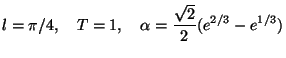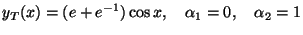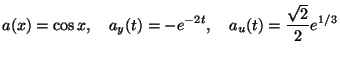(5.1)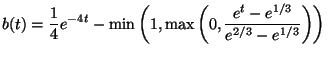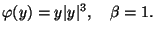As is shown in  a local optimum for this problem is the pair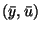,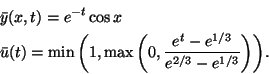The discretization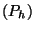of section 2 was coded in AMPL and solved with LOQO. Afterwards, the SSC were checked as described in the previous section resulting in the minimal eigenvalue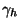, cf. (4.3).

Table 1: Solution errors for problem 5.1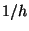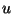-error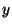-error 100 7.444e-5 1.208e-5 200 1.884e-5 3.128e-6 300 9.279e-6 1.412e-6

Table 2: Minimal eigenvalue for problem 5.1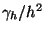100 1.774e-4 1.77 200 4.266e-5 1.71 300 1.923e-5 1.73

In Table 1 the maximum errors over the grid points are listed for the computed control and state functions while in Table 2 the smallest eigenvalue is listed as well as the value scaled by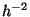. The errors exhibit quadratic convergence while the scaled eigenvalue stays nearly constant with a slightly increasing tendency. These facts justify the application of the technique to other control problems for which no exact solution is known and it also shows which scaling ofis appropriate for problem. This scaling is also suggested by known quadratic convergence estimates for fixed control in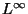since the differential equation as well as all boundary conditions were discretized with second-order consistency. An argument could be made that the smallness of the eigenvalue may indicate that it is, in fact, negative and the relatively coarse discretization prevents it from exhibiting this fact. This seems highly unlikely in the light of the very uniform and even slightly growing behavior of the scaled value combined with the fact that all the eigenvalues were computed and the smallest one is not considerably smaller than the next ones but they grow only gradually.

As a second parabolic case one from  was chosen. The data for (P) are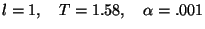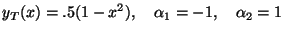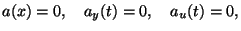(5.2)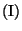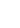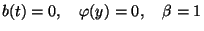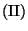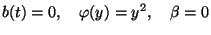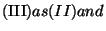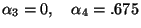The case (I) leads to a linear-quadratic control problem and had already been considered in . Here, both lower and upper control bounds are active in the solution while for (II) the lower control bounds are active only, and for (III) additionally the lower and upper state constraints. For all cases just the minimal eigenvalue is listed without and with the same scaling as in the previous example.

Table 3: Minimal eigenvalue for 5.2-I100 1.908e-6 1.91e-2 200 4.726e-6 1.89e-2

Table 4: Minimal eigenvalue for 5.2-II100 9.431e-7 9.43e-3 200 2.447e-7 9.79e-3

Table 5: Minimal eigenvalue for 5.2-III100 1.010e-6 1.01e-2 200 2.611e-7 1.04e-2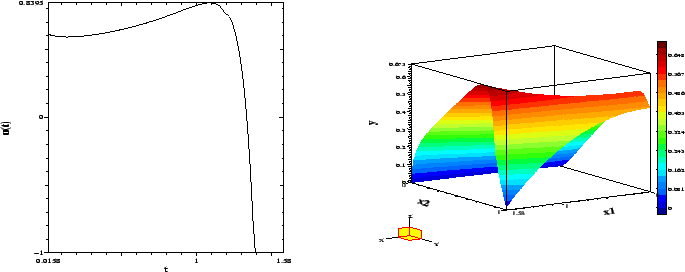We include a plot of the optimal control and state for this problem. Due to the consistent results obtained the computations for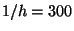were not done in these cases.
Finally, we solve a boundary control problem for the instationary Burgers equation, see also . For simplicity we used the same data as for case (II) above with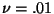and the control bounds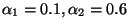both of which become active in the solution. This case is denoted by 5.2-IV. It clearly shows that for this problem the suitable normalization of the eigenvalue is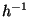. This will have to be confirmed by a careful comparison of the continuous and discrete SSC as had been done for the first order necessary conditions in [16,17]. A similar situation arose in the elliptic boundary control problem in  which is addressed next below. For it in  the scaling byseemed appropriate in contrast to the other such problems considered.

Table 6: Minimal eigenvalue for 5.2-IV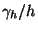100 1.211e-5 1.21e-3 200 5.939e-6 1.19e-3

Next, we present the data for the elliptic boundary control problem from  and the eigenvalues obtained. The domain is the unit square. The problem (EB) together with (2.1) and (2.2) is considered and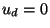.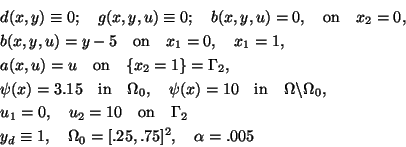Table 7: Minimal eigenvalue for (EB)120 1.472e-5 2.12e-1 180 6.525e-6 2.11e-1

We also include a plot of the optimal control and state for this problem. For plots of the adjoint variables and further details, we refer to .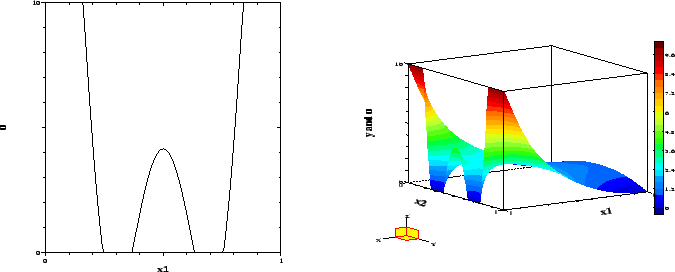Next: Conclusion Up: Sufficient Optimality for Discretized Previous: Second Order Sufficient Conditions
Hans Mittelmann
2000-08-31